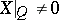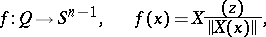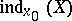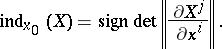# Singular point, index of a

(diff) ← Older revision | Latest revision (diff) | Newer revision → (diff)

One of the basic characteristics of an isolated singular point of a vector field. Let a vector fieldbe defined on, and letbe a sphere of small radius surrounding a singular pointsuch that. The degree of the mapping (cf. Degree of a mapping)is then called the index,, of the singular pointof the vector field, i.e.Ifis non-degenerate, then